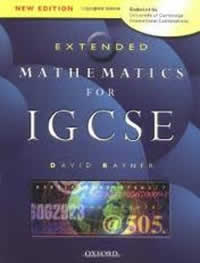# Maths Tutors & Maths Tuition – Find a private Maths Tutor in Gurgaon

The Expert Maths Tutor for Students of All Ages It’s difficult to know where weaknesses in maths started. Ideas in maths build on one another so gaps in knowledge can mean future lessons don’t make sense and confidence drops. Everyone learns at a different pace. A really important factor with maths learning is consistency… maths…Continue reading Maths Tutors & Maths Tuition – Find a private Maths Tutor in Gurgaon